# Exercise E5.1 Introduction to Euclids Geometry NCERT Solutions Class 9

Go back to  'Introduction to Euclids Geometry'

## Chapter 5 Ex.5.1 Question 1

Which of the following statements are true and which are false? Give reasons for your answers.

(i) Only one line can pass through a single point.

(ii) There are an infinite number of lines which pass through two distinct points.

(iii) A terminated line can be produced indefinitely on both the sides.

(iv) If two circles are equal, then their radii are equal.

(v) In figure 5.9  if

$$AB = PQ$$ and $$PQ = XY,$$

then $$AB = XY.$$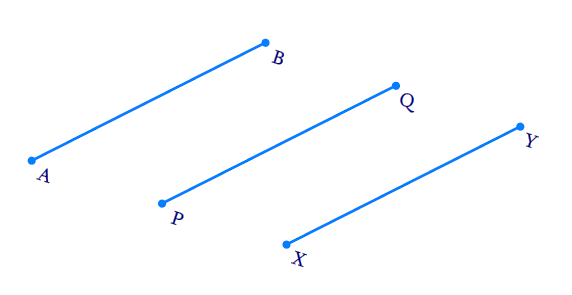Figure 5.9

### Solution

(i)  Reasoning:

We can draw infinite number of lines through a given point.

Steps:

False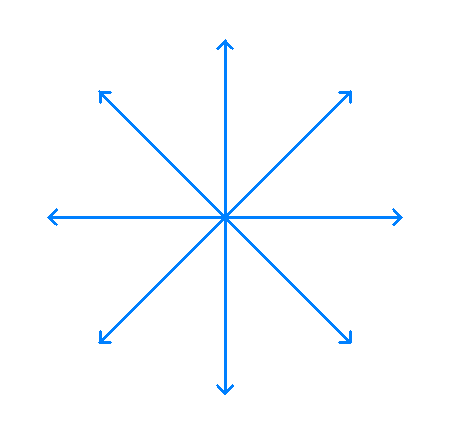Related problems:

1. What are concurrent lines?
2. How many chords can be drawn though center of the circle?

(ii) Reasoning:

According to Axiom 5.1: Given any two distinct points, there is a unique line that passes through them.

Steps:

False

We can draw only one line passing through two points.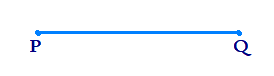(iii)  Reasoning:

According to Postulate 2 :A terminated line can be produced indefinitely.

Steps:

True

We know that a straight line can be produced on both sides.(vi)  Reasoning:

According to Postulate 3: A circle can be drawn with any center and any radius.

Steps:

True

We know that circles are equal, means the circles are congruent. (Circles coinciding with each other). This means that circumferences are equal and so the radii of two circles are equal.

(v)  Reasoning:

Line segments whose corresponding lengths are equal are equal to one another.Steps:

True

By transitivity law, we know that, if $$a = b$$ and $$b = c$$ then $$a = c.$$

Here since $$AB = PQ$$and $$PQ = XY$$ then $$AB = XY$$ according to this law.

Let us consider $$AB =5 \;\rm{cm}$$, then $$PQ$$ will be $$5 \;\rm{cm}.$$

But $$PQ = XY$$ so $$XY$$ will also be $$5 \;\rm{cm}$$. $$AB = PQ = XY$$ which also implies $$AB = XY.$$

## Chapter 5 Ex.5.1 Question 2

Give a definition for each of the following terms. Are there other terms that need to be defined first? What are they, and how might you define them?

(i) Parallel lines

(ii) Perpendicular lines

(iii) Line Segment

(v) Square

### Solution

Steps:

We have to define ‘Ray’, ‘Straight line’ and a ‘point’.

Ray: A part of a line, which starts at a point (Here A)and goes off in a particular direction to infinity possibly through a second point(B in this case).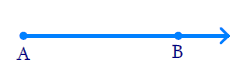Straight Line: The basic concept about a line is that it should be straight and it can be extended infinitely in both the directions.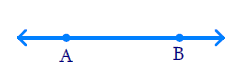Point: A small dot made by a sharp pencil on a sheet paper gives an idea about a point. A point has no dimension, it has only a position.

Perpendicular Distance: Shortest distance between a point and a line.

(i) Parallel lines:

• If the perpendicular distance between two lines is always constant, then these are called parallel lines.
• If the lengths of the common perpendiculars at different points on the lines are same, then these lines are called parallel lines.
• In other words, the lines which never intersect each other are called parallel lines.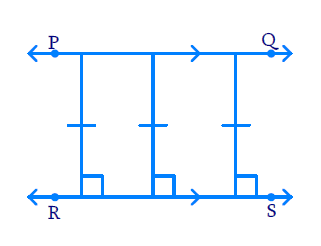(ii) Perpendicular lines: If the angle between two lines is equal to , then these lines are perpendicular to each other.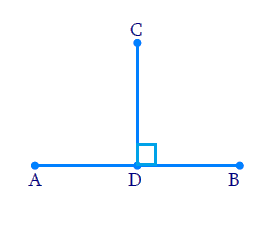\begin{align} { C D \perp A B } \\ { A B \perp C D } \end{align}

(iii) Line segment: A terminated line is called line segment. It has two end points [A and B in this case.].(iv) Radius of a circle: The distance from the center to any point on the circle is called radius of the circle.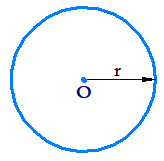(v) Square: A square is a regular quadrilateral which means that it has four equal sides and four right angles.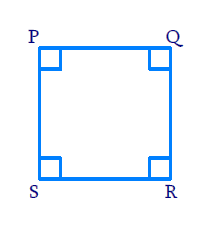## Chapter 5 Ex.5.1 Question 3

Consider two ‘postulates’ given below:

(i) Given any two distinct points $$A$$ and $$B$$ there exists a third point $$C$$ which is in between $$A$$ and $$B$$.

(ii) There exist at least three points that are not on the same line.

Do these postulates contain any undefined terms? Are these postulates consistent? Do they follow from Euclid’s postulates? Explain.

### Solution

#### Reasoning:

Euclid’s axioms.

Steps:

Yes, these postulates contain undefined terms. These two statements are consistent as they talk about two different situations.

These statements do not follow Euclid’s postulates but one of the axiom about “Given any two points, a unique line that passes through them” is followed.

## Chapter 5 Ex.5.1 Question 4

If a point $$C$$ lies between two points $$A$$ and $$B$$ such that $$AC = BC$$, then prove that

\begin{align}AC = \frac{1}{2}AB.\end{align} Explain by drawing the figure.

### Solution

Reasoning:

We are aware that when equals are added to equals, the wholes are equal.

#### Steps: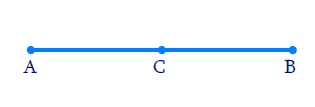$AC =BC$

Adding $$AC$$ on both sides, we get

\begin{align}&AC+AC=BC+AC \\ &2AC=AB \\\\& \left( \text{BC}\text{+}\text{AC}\,\,\text{coincides with}\text{AB} \right) \\&AC=\frac{1}{2}AB \\ \end{align}

## Chapter 5 Ex.5.1 Question 5

In a Question 4, point $$C$$ is called a mid-point of line segment $$AB$$. Prove that every line segment has one and only one mid-point.

### Solution

Reasoning:

We are aware that the things which coincide with one another are equal to one another.

Steps:

Let us consider that line segment $$AB$$ has two mid points $$‘C’$$ and $$‘D’$$.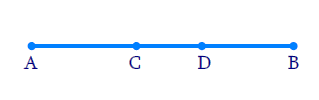Let assume $$C$$ is mid-point of $$AB$$

$AC = BC$

Adding $$AC$$ on both sides, we get

\begin{align} AC+AC & = BC+AC \\ 2 AC & = AB \\ AC& = \frac { 1 } { 2 } AB \ldots \ldots ( 1 ) \end{align}

Let us consider a point $$D$$ lying on $$AB,$$ Let assume that $$D$$ be another mid-point of $$AB.$$

Therefore $$AD = BD$$ Adding equal length $$AD$$ on both the sides, we get

\begin{align} AD+AD & = BD+AD \\ 2 AD & = AB \\ AD & = \frac { 1 } { 2 } AB \ldots \ldots ( 2 ) \end{align}

From equations ($$1$$) and ($$2$$), we can conclude that $$AC = AD$$

• $$C$$ Coincides with $$D.$$
• Axiom $$4$$: Things which coincide with one another are equal to one another.
• A line segment has only one midpoint.

## Chapter 5 Ex.5.1 Question 6

In the given figure, if $$AC = BD$$ then prove that $$AB = CD.$$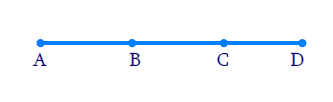### Solution

Reasoning:

We know that when equals are subtracted from equals, the remainders are equal.

Steps:

\begin{align} AC & = BD \\ AB + BC & = BC + CD \end{align}

[ Point $$B$$  lies between $$A$$ and $$C$$ point $$C$$ lies between $$B$$ and  $$D$$.]

\begin{align} AB + BC - BC & = BC + CD - BC \\ AB & = CD\end{align}

## Chapter 5 Ex.5.1 Question 7

Why is Axiom $$5$$, in the list of Euclid’s axioms, considered a ‘universal truth’? (Note that the question is not about the fifth postulate.)

### Solution

Reasoning:

Axiom $$5$$ – “The whole is greater than the part.”

steps:

This axiom is known as a universal truth because it holds true in any field of mathematics and in other disciplinarians of science as well.

i). Let us consider a line segment $$AB$$. Mark two points $$P$$ and $$Q$$ on it.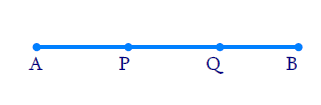$$AB$$ is a whole part. It is divided into three parts: $$AP,\;PQ,\;QB.$$

\begin{align} A B &= A P + P Q + Q B \\ A B &> A P \\ A B &> P Q \\ A B &> Q B \end{align}

ii). Bangalore is a part of Karnataka which means that Karnataka is larger than Bangalore. i.e. Karnataka $$>$$ Bangalore.

Therefore, it is true that the whole is greater than the part is considered as universal truth.

Related Sections
Related Sections